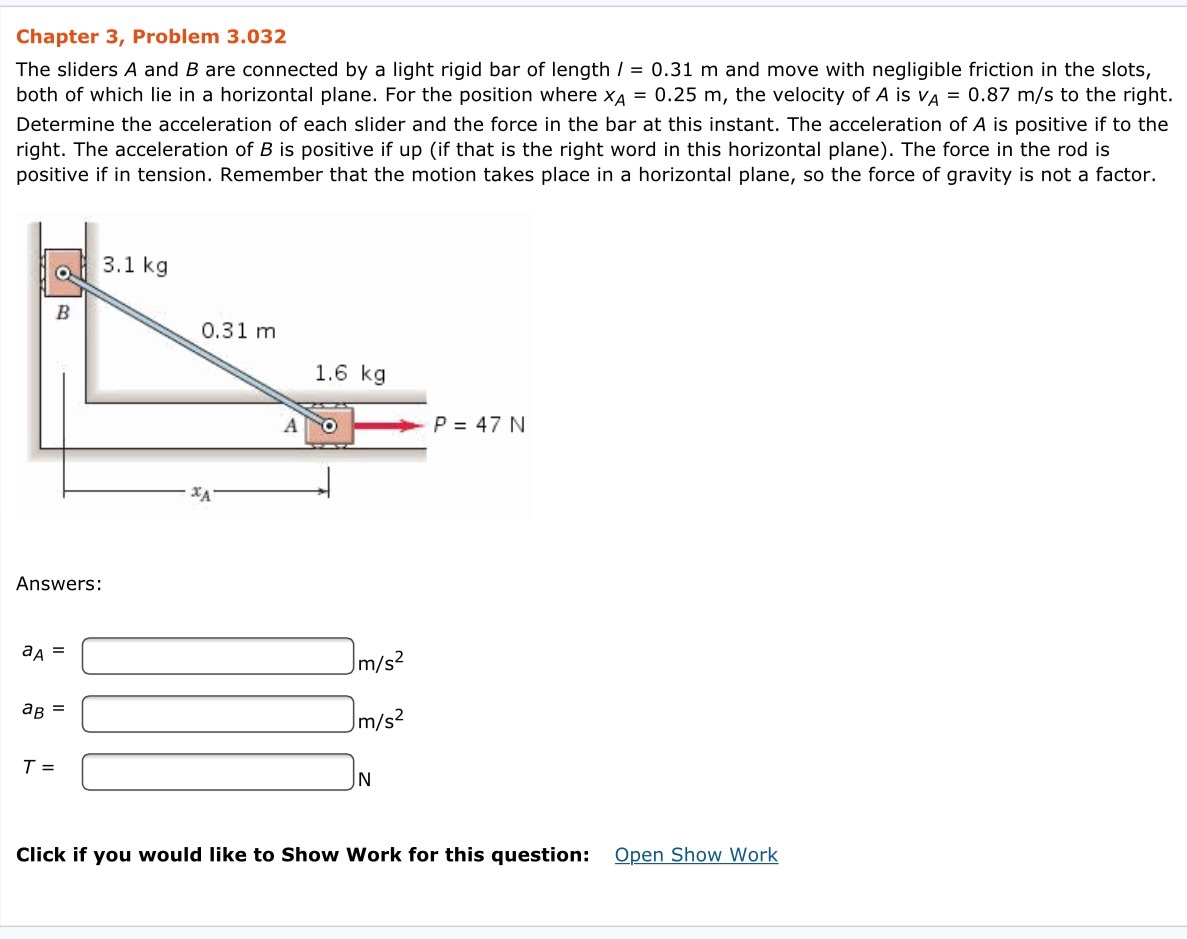### Create an Account

Home / Questions / The sliders A and B are connected by a light rigid bar of length l 031 m and move with ne...

# The sliders A and B are connected by a light rigid bar of length l 031 m and move with negligible friction in the slots both of which lie in a horizontal plane For the position where

 The sliders A and B are connected by a light rigid bar of length l = 0.31 m and move with negligible friction in the slots, both of which lie in a horizontal plane. For the position where xA = 0.25 m, the velocity of A is vA = 0.87 m/s to the right. Determine the acceleration of each slider and the force in the bar at this instant. The acceleration of A is positive if to the right. The acceleration of B is positive if up (if that is the right word in this horizontal plane). The force in the rod is positive if in tension. Remember that the motion takes place in a horizontal plane, so the force of gravity is not a factor.View less »

Jun 19 2020 View more View LessSubscribe To Get Solution